Technical Report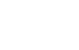Order ratio analysis and tracking analysis
(page2)

2. Rotation-tracking analysis

2-1 Constant ratio tracking analysis

Using a rotary pulse as the sampling clock of the FFT analyzer, which normalized by rotational order rather than frequency on the horizontal axis is order ratio analysis as mentioned in the previous section and as shown in Figure a as below.

Constant ratio tracking analysis of rotation-tracking analysis is a tracking analysis that follows the level change of the order component according to the rotation speed by specifying an arbitrary component of order components, changing the rotation speed as shown in rotation speed- spectral diagram in Figure b below. It is displayed in Figure c.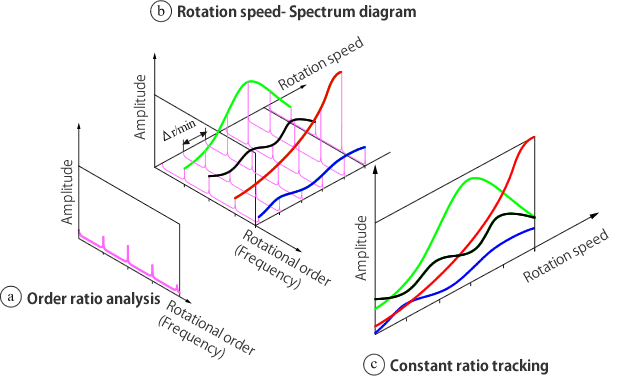Fig.6

Fig. 7 below shows an example of the first, second, third, and fourth-order of constant ratio tracking analysis of for noise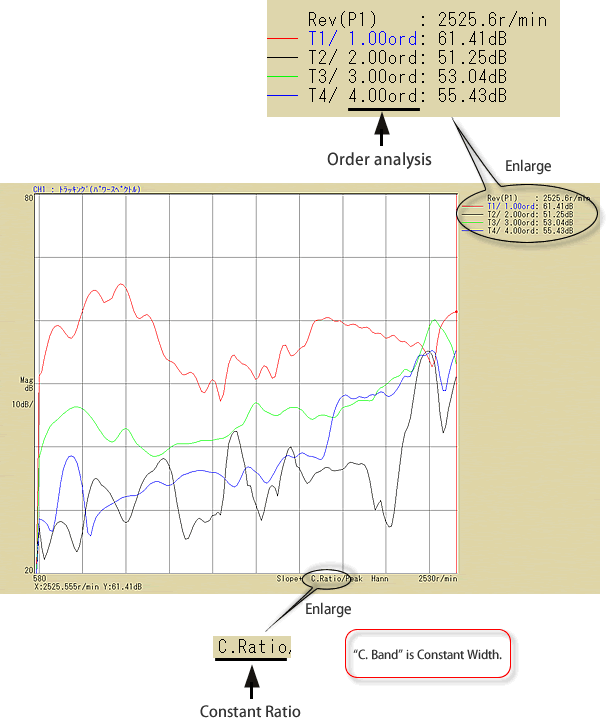Fig７

2-2 Constant width tracking analysis

Constant width tracking analysis is shown in Figure 8. By using the internal sampling clock of FFT analyzer, extracting arbitrary order components from frequency analysis data displayed in three-dimensional, and plotting, tracking analysis is performed.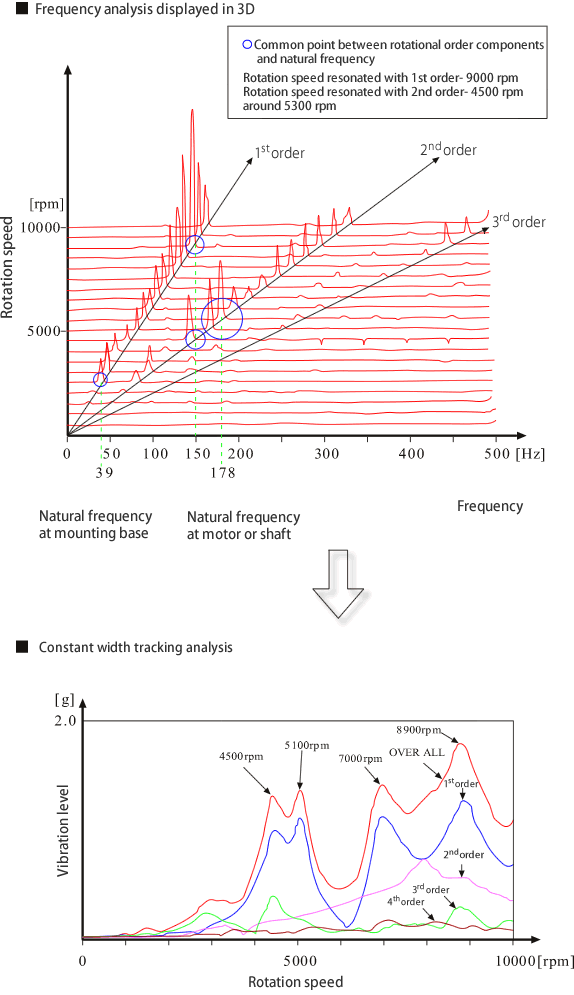Fig.8

2-3 Other tracking analysis

There are other tracking analyses other than constant ratio, constant width as below. The analysis method is same as constant width.

• Constant width frequency tracking

Tracking a specified frequency component using internal sampling clock according to rotation speed. This is not order ratio analysis.

• Octave tracking

Same as constant width frequency tracking, but tracking a specified band component in 1/3, 1/1 octave band data according to rotation speed.

2-4 Tracking analysis and rotation change rate

When tracking analysis is performed, you may change rotation speed accordingly. If the rotation speed changes too fast with respect to the analysis operation time, the waveform of the spectrum will be shifted, and correct analysis cannot be performed. When order analysis is performed by increasing the rotation speed, the results of spectral analysis based on the difference in rotation change rate is shown in Figure 9 below. When rotation change rate is small, analysis is performed properly in Fig 9-a. When rotation change rate is large, the peak of the spectrum is shifted from the original order position to the smaller one in Fig 9-b. When rotation change rate is large, the base of peak extends in the lower order direction in Fig. 9-c. It is important to perform this test after operating at the scheduled rotation speed change and confirming that the order ratio analysis and frequency analysis data are as shown in Figure 9-a.

 • Rotation change rate is small.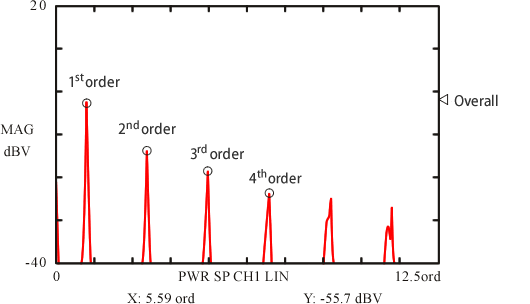fig. 9-a • Rotation change rate is large.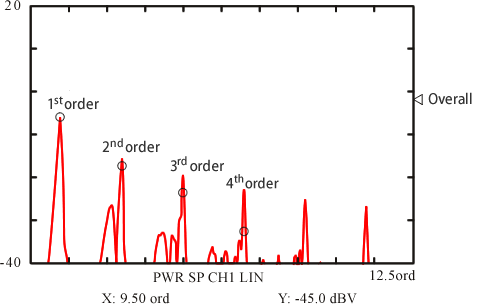Fig. 9-b • Rotation change rate is large.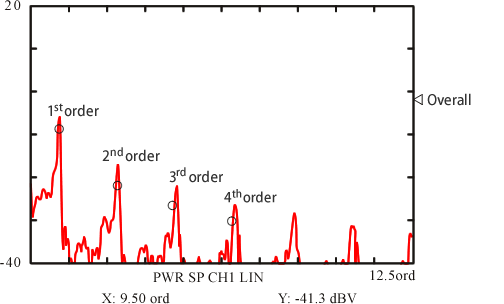Fig. 9-c

3. Summary

Here is a summary of what we have explained so far, comparing constant ratio order tracking and constant width order tracking, which are the main of tracking analyses.

3-1 Constant ratio order tracking and Constant width order tracking

Constant ratio order tracking - Tracking analysis is performed by using the rotation pulses obtained from the rotating body as an external sampling clock. It plots the changes in the spectrum level of the specified order component corresponding to rotation speed.
Constant width order tracking- Frequency analysis is performed via internal sampling clock. Each time rotation speed changes, frequency of order is measured from frequency range and rotation speed at that time. A plot is created for the changes in the spectrum level of this frequency component corresponding to the changes in rotation speed.
In constant ratio tracking analysis and order ratio analysis, when viewing from order axis, the maximum analysis order and order resolution (order bandwidth) are constant regardless of the rotation speed. When viewing from frequency axis, the maximum analysis order and order resolution (order bandwidth) vary according to the change of rotation speed as in the left of Figure 10. For example, when amplitude is analyzed by the certain random signal, order component level is larger, the rotation speed is higher. This tendency is more particular in overalls since the frequency range is widened. It is shown in the right of Figure 10.
In constant width tracking analysis and frequency analysis, when viewing from frequency axis, the maximum analysis order and order resolution (order bandwidth) are constant based on frequency range regardless of the rotation speed. Please see Figure 10 vs Figure 11 as follow.
Even if the rotation speed changes, frequency bandwidth, overall frequency range corresponding to the order are constant. That is, when rotation speed is low, order bandwidth is wide, and when rotation speed is high, order bandwidth is narrow. In other words, in constant width order tracking analysis, the level of order component tends to decrease as rotation speed increases, compared to constant ratio tracking analysis. It is a bit complicated as there is a conflicting relationship between order ratio analysis and frequency analysis. It is necessary to understand the characteristics of each analysis type.

• Constant ratio order tracking analysis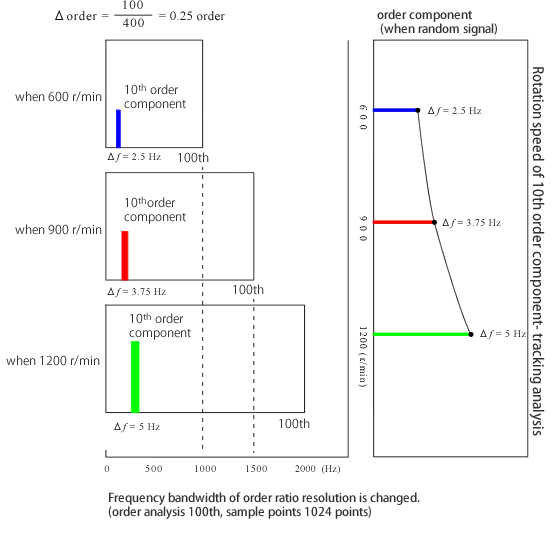Frequency f of 100th order at 600 r/min;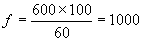Frequency bandwidth of each order components Δf ;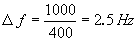Frequency f of 100th order at 1200 r/min,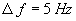Fig. 10

•Constant width order tracking analysis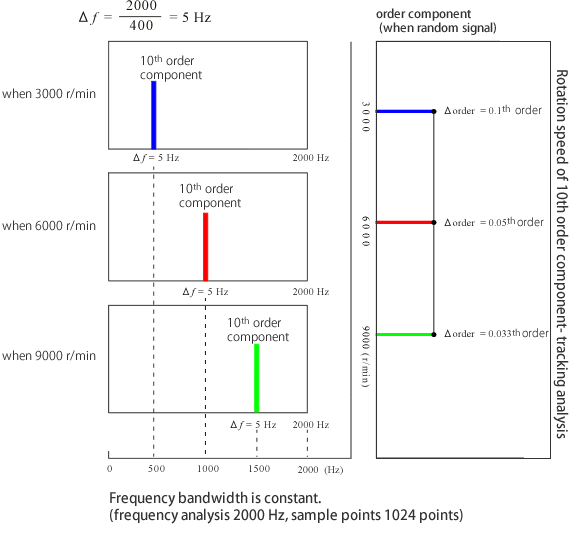Frequency f of 100th order at 3000 r/min；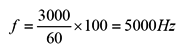Δ order at 3000 r/min = Frequency of 1st order Δf ；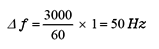When Δf = 5 Hz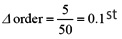When 9000 r/min, Δ order = Approx. 0.033th

Fig. 11

Notes for performing Constant ratio order tracking and constant width order tracking analysis are as follow.

Constant ratio order tracking Constant width order tracking
Order analysis resolution is fixed regardless of rotation speed.
In the case of the signals resembling random noise without any clear peak as order component, the frequency bandwidth (resolution) becomes high at the higher rotation speed. Therefore, there is a tendency of the spectrum numerical value being large.

Frequency resolution is fixed regardless rotation speed.

When frequency range is low, it will be difficult to increase rotation speed ratio higher as constant ratio order tracking.
It is necessary to determine the maximum frequency before setting the number of analysis order (because the upper limit frequency is limited by setting the frequency range).